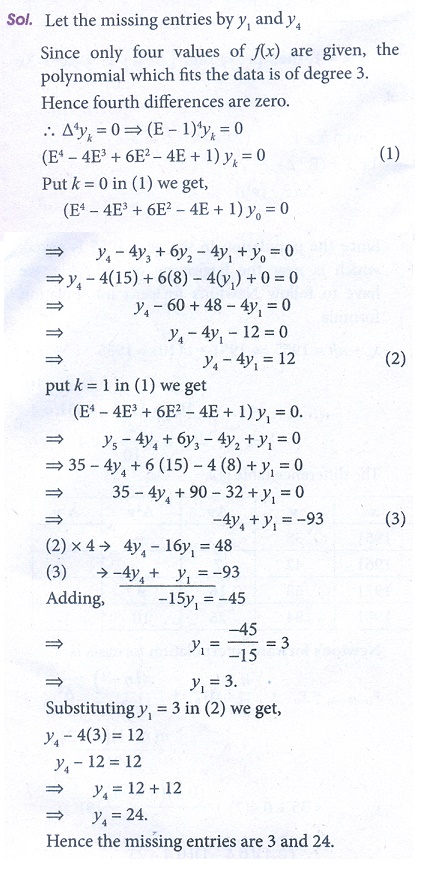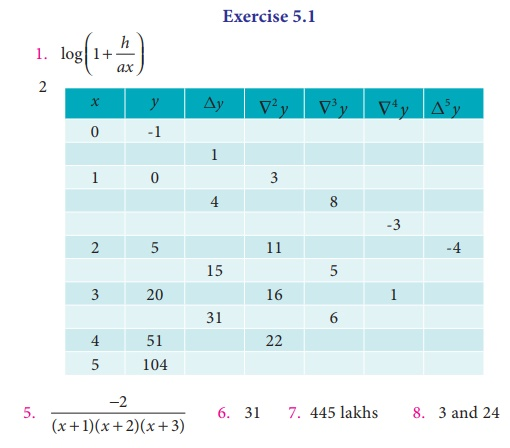Home | | Business Maths 12th Std | Exercise 5.1: Finite Differences

# Exercise 5.1: Finite Differences

Book back answers and solution for Exercise questions - Maths: Numerical Methods: Finite Differences

Exercise 5.1

1. Evaluate ╬ö(log ax) .2. If y = x3 ŌłÆ x2 + x ŌłÆ1 calculate the values of y for x = 0,1,2,3,4,5 and form the forward differences table.3. If h = 1 then prove that (EŌłÆ1 ╬ö)x3 = 3x2 ŌłÆ 3x + 1 .4. If f (x= x2 + 3x than show that ╬ö f (x= 2x + 45. Evaluate ╬ö [1 /  (x + 1)( x + 2 ) ] by taking ŌĆś1ŌĆÖ as the interval of differencing6. Find the missing entry in the following table7. Following are the population of a districtFind the population of the year 19118. Find the missing entries from the following.